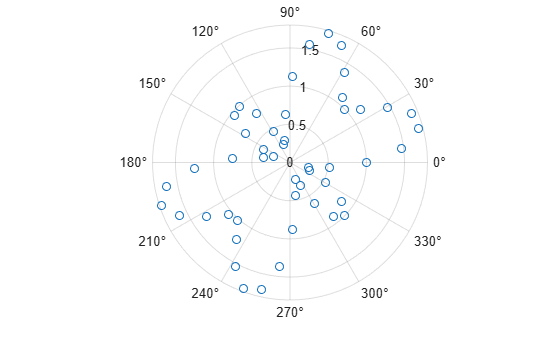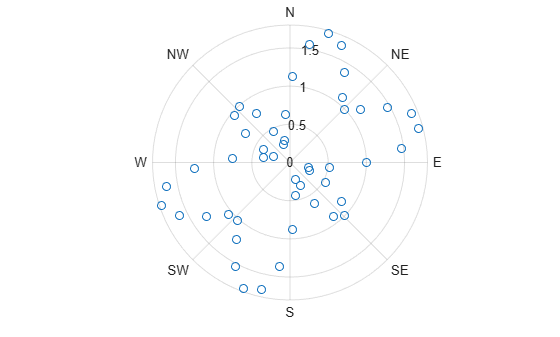Compass Labels on Polar Axes

This example shows how to plot data in polar coordinates. It also shows how to specify the angles at which to draw grid lines and how to specify the labels.

Plot data in polar coordinates and display a circle marker at each data point.

theta = linspace(0,2*pi,50);
rho = 1 + sin(4*theta).*cos(2*theta);
polarplot(theta,rho,'o')Use gca to access the polar axes object. Specify the angles at which to draw grid lines by setting the ThetaTick property. Then, specify the label for each grid line by setting the ThetaTickLabel property.

pax = gca;
angles = 0:45:360;
pax.ThetaTick = angles;
labels = {'E','NE','N','NW','W','SW','S','SE'};
pax.ThetaTickLabel = labels;